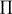### PROD Aggregation Expression

PROD { index-set } expression

The PROD aggregation operator evaluates the numeric expression expression for each member of the index set index-set. The result is the product of the values that are returned by the expression. This operator is analogous to theoperator used in mathematical notation. If the index set is empty, then the result is 1.

The following example uses the PROD operator to evaluate a factorial:

```proc optmodel;
number n = 5;
put (prod{i in 1..n} i); /* outputs 120 */
```